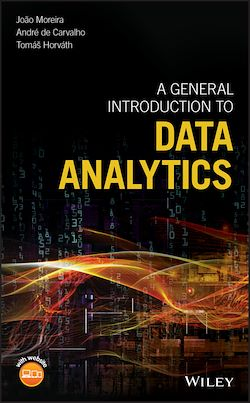352 Pages
English

# A General Introduction to Data Analytics

-Description

A guide to the principles and methods of data analysis that does not require knowledge of statistics or programming

A General Introduction to Data Analytics is an essential guide to understand and use data analytics. This book is written using easy-to-understand terms and does not require familiarity with statistics or programming. The authors—noted experts in the field—highlight an explanation of the intuition behind the basic data analytics techniques. The text also contains exercises and illustrative examples.

Thought to be easily accessible to non-experts, the book provides motivation to the necessity of analyzing data. It explains how to visualize and summarize data, and how to find natural groups and frequent patterns in a dataset. The book also explores predictive tasks, be them classification or regression. Finally, the book discusses popular data analytic applications, like mining the web, information retrieval, social network analysis, working with text, and recommender systems. The learning resources offer:

• A guide to the reasoning behind data mining techniques
• A unique illustrative example that extends throughout all the chapters
• Exercises at the end of each chapter and larger projects at the end of each of the text’s two main parts

Together with these learning resources, the book can be used in a 13-week course guide, one chapter per course topic.

The book was written in a format that allows the understanding of the main data analytics concepts by non-mathematicians, non-statisticians and non-computer scientists interested in getting an introduction to data science. A General Introduction to Data Analytics is a basic guide to data analytics written in highly accessible terms.

Subjects

##### Classwork

Informations